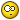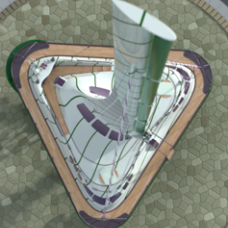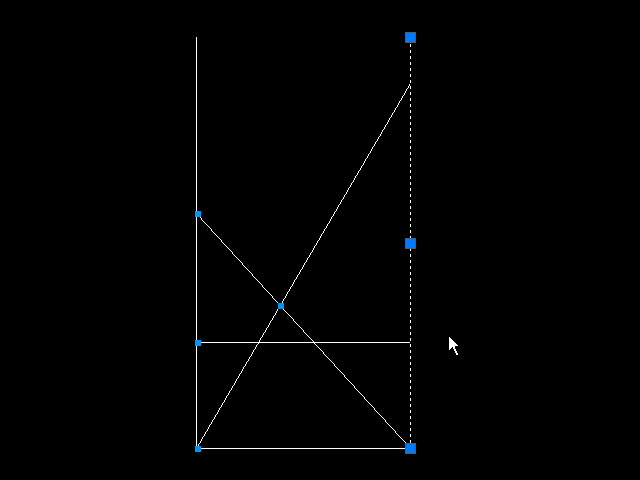# Can ACAD figure out the angle to put a line of specific length between parallel lines## Recommended PostsI hope I can explain this simply enough:I am drawing a diagram to describe a mathematical word problem. Since it is easier to visualize with a drawing, I want to draw it using the given dimensions. But angles used in the diagram are unknown and are not needed for the answer to be calculated, so the only way to draw this freehand is to guess at the angle resulting in the correct distances to be off.

Here is the word problem:

There are two ladders, one 40 feet and the other 30 feet, each touching the base of one of two buildings and leaning against the other building. If the ladders cross 10 feet above the ground, how far apart are the buildings?

So for my drawing, being rather simple considering what AutoCAD can do, results in this:

Can AutoCAD calculate the angle needed to place a line of a given length between two parallel lines that are a distance apart less than the length of the line to place between them, having the endpoints of this line touching the parallel lines, fitting exactly?

For the word problem, the distance between the two parallel lines is what needs to be solved and the math is quite invloved, having to find the roots or solution of a quartic equation. (I have the solution figured out to be 26.03287754 feet)

What I am afraid of is that this would require to program an AutoLISP routine to create a new command that one can call from the command line. I see it using the the measure command with relative and absolute co-ordinates. This may be far from a "beginner" question. I can do this quite easily on a peice of paper: having two parallel lines drawn, take a ruler and placing the "0" mark on one line, then adjusting the angle until the desired length just reaches the opposite parallel line. I used to use this trick quite often when drafting with pencil and paper to divide a distance into a required number of even amounts, so this can't be that difficult to do with AutoCAD!

If anyone needs further details just let me know!

##### Share on other sitesTypically, this is done with construction geometry. A circle, of radius 40, say, could be centered on one endpoint. A line can then be drawn from that endpoint to the intersection of the circle and the other line.

Another option would be the use of geometric constraints.

##### Share on other sitesHave I missed the point? Since you actually know the distance apart of the the two parallel lines and the two lengths you have every thing necessary to draw it. (I too would use a circle of the given radii to find the intersection points.)

##### Share on other sitesYes, You missed the point. The problem doesn't state how far apart the buildings are. The object of the problem is to determine how far apart they are.

The problem states a 30ft ladder and a 40ft ladder placed at the base of one building and leaning accost to the other building, cross ten feet above the ground. How far apart are the buildings. .

Clearly, that is the word problem.

The problem related to the original post, though, may not be so clear. Based on the wording in the second and second to last paragraphs, it sounds like the requirement is a drafting solution to help illustrate the word problem.

##### Share on other sitesI accept I have missed the point but I have also not understood the question. At first I assumed one ladder was against one building, the other laadder against the other building but now it appears they are against the same building in which case I cannot visualise how they could possibly cross.

##### Share on other sitesI deleted my original post I miss read your statement. You are correct the ladders are at the base of one building and leaning across the the other crossing at ten feet above the ground.

##### Share on other sitesLines originating from the same point will never intersect, so the ladders must be opposing each other despite the wording.

##### Share on other sitesCan AutoCAD calculate the angle needed to place a line of a given length between two parallel lines that are a distance apart less than the length of the line to place between them, having the endpoints of this line touching the parallel lines, fitting exactly?

Since you are using 2012 - simply use parametrics. (see the Parameters tab)

Fix constrain endpoints or add a parametric aligned dimension between the two.

Add the line at eyeball angle (any angle will do)

Add the parametric dimension for the length of the line - AutoCAD will automatically resize it at the only possible solution.

This demonstrates the real power of parametric dimensions and geometric constraints (not to be confused with osnaps).

I just went back and read the actual word problem (I hadn't really paid attention to the problem description) but that is how I would set it up - using AutoCAD parametrics, just make sure you use the logical parameters. If you (or someone) doesn't come back with the actual parametric solution, I'll come back and take a closer look. Take a shot at it and see if you can figure out the process.

My daughter was home-schooled.

I used this to teach her trig in a visual way.

I could drag a triangle and show sine and cosine values graphically and interactively.

She started college at age 14.

Edited by JD Mather
##### Share on other sitesGeometric/Parametric constraints..... I'm actually not sure how I'd do it in AutoCAD without them. For demonstration purposes, I omitted the constraint locking the intersection at 10' elevation.##### Share on other sitesI would comment that climbing the 30' ladder would be very bouncy

##### Share on other sitesNow that I’ve re-read the word problem, perhaps it's not asclear as I first thought. I definitely picturethe layout nestly has animated above.

## Join the conversation

You can post now and register later. If you have an account, sign in now to post with your account.
Note: Your post will require moderator approval before it will be visible.×   Pasted as rich text.   Restore formatting

Only 75 emoji are allowed.